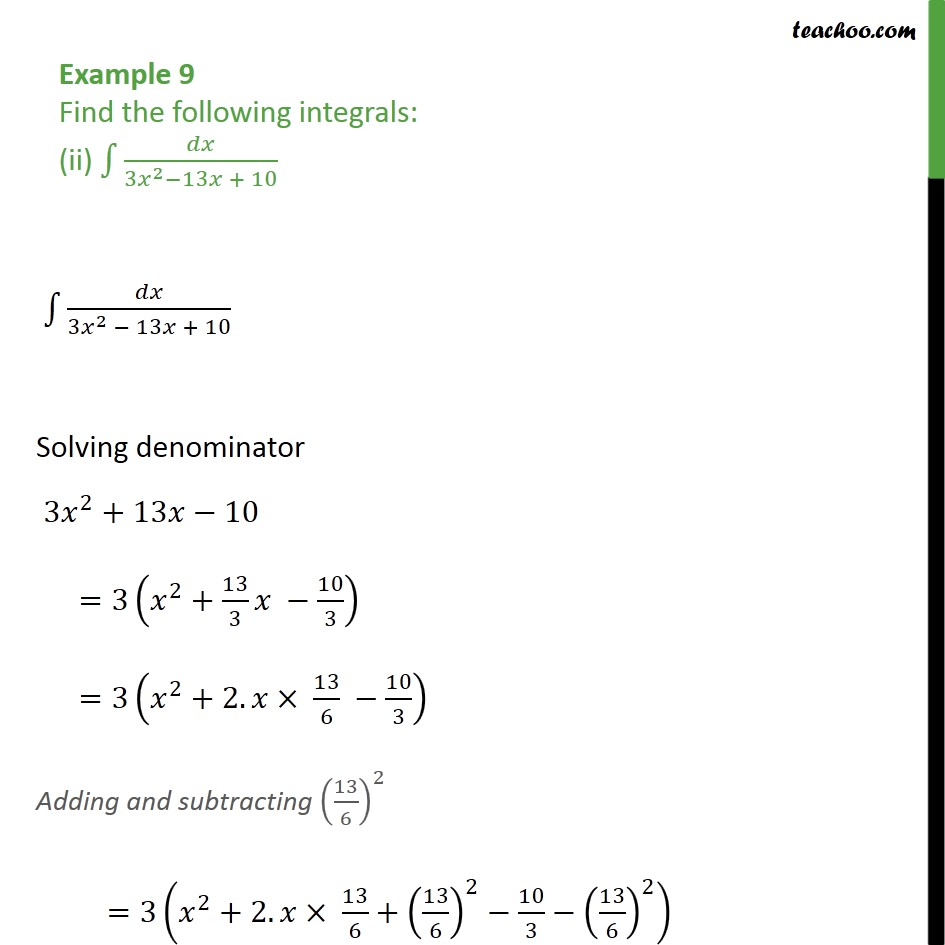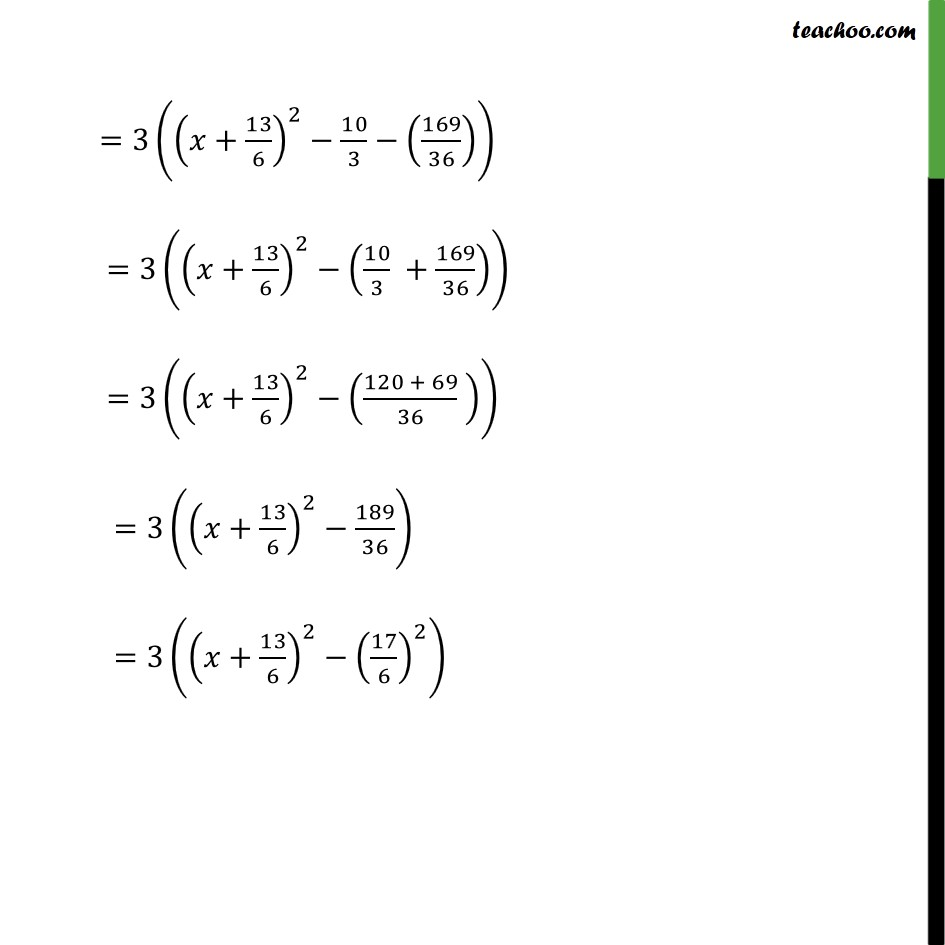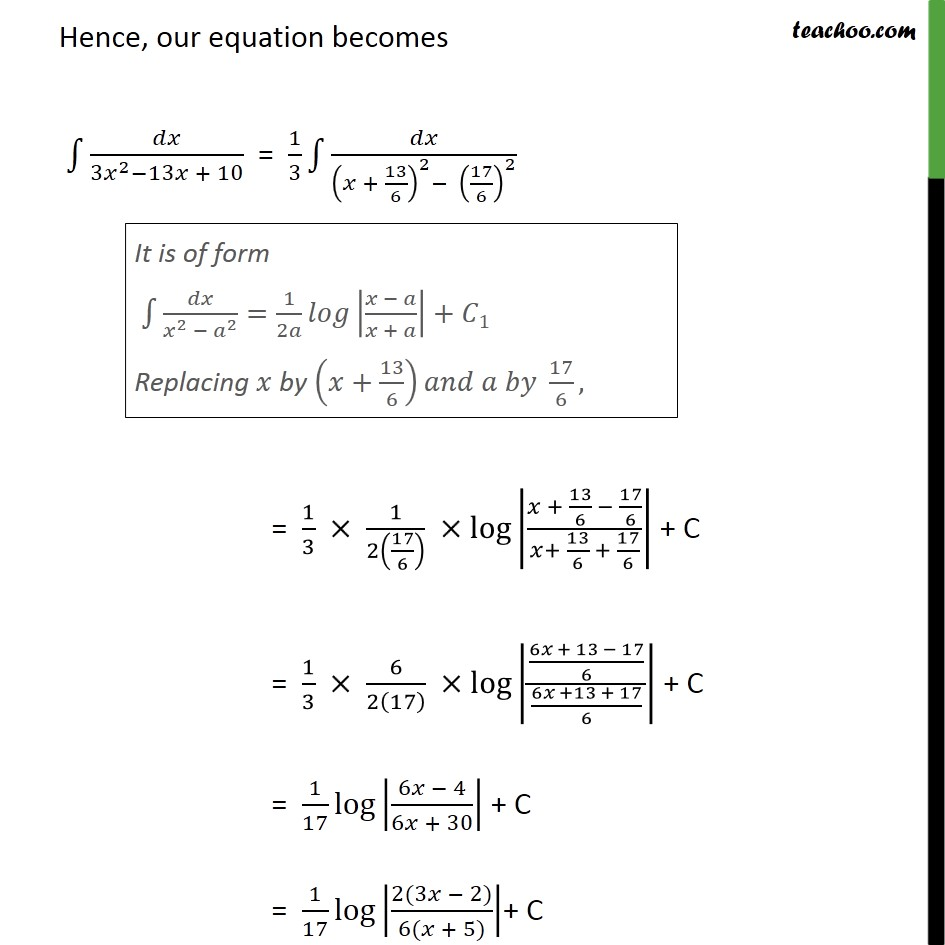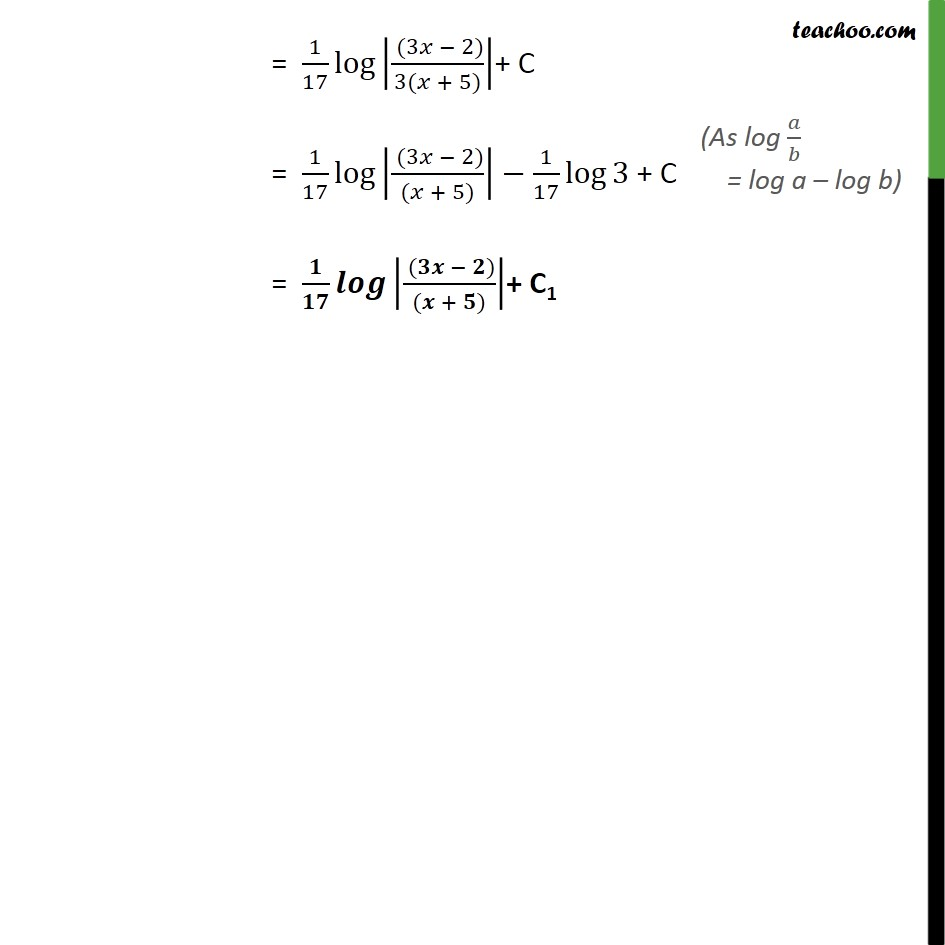Integration by specific formulaes - Formula 1

Chapter 7 Class 12 Integrals
Concept wiseLearn in your speed, with individual attention - Teachoo Maths 1-on-1 Class

### Transcript

Example 9 Find the following integrals: (ii) 3 2 13 + 10 3 2 13 + 10 Solving denominator 3 2 +13 10 =3 2 + 13 3 10 3 =3 2 +2. 13 6 10 3 Adding and subtracting 13 6 2 =3 2 +2. 13 6 + 13 6 2 10 3 13 6 2 =3 + 13 6 2 10 3 169 36 =3 + 13 6 2 10 3 + 169 36 =3 + 13 6 2 120 + 69 36 =3 + 13 6 2 189 36 =3 + 13 6 2 17 6 2 Hence, our equation becomes 3 2 13 + 10 = 1 3 + 13 6 2 17 6 2 = 1 3 1 2 17 6 log + 13 6 17 6 + 13 6 + 17 6 + C = 1 3 6 2 17 log 6 + 13 17 6 6 +13 + 17 6 + C = 1 17 log 6 4 6 + 30 + C = 1 17 log 2(3 2) 6( + 5) + C = 1 17 log 2(3 2) 6( + 5) + C = 1 17 log (3 2) 3( + 5) + C = 1 17 log (3 2) ( + 5) 1 17 log 3 + C = ( ) ( + ) + C1